# How To Do Long Division

Turn your big numbers into smaller ones with long division – just master these easy steps.Author
Taylor HartleyExpert Reviewer

Published: August 24, 2023# How To Do Long Division

Turn your big numbers into smaller ones with long division – just master these easy steps.Author
Taylor HartleyExpert Reviewer

Published: August 24, 2023# How To Do Long Division

Turn your big numbers into smaller ones with long division – just master these easy steps.Author
Taylor HartleyExpert Reviewer

Published: August 24, 2023Key takeaways

• Long division is a process for dividing large numbers – This method breaks dividing large numbers into a sequence of easy steps you can use to find the right answer.
• It’s different from the short division method – Long division is primarily used with dividends of two or more digits. This makes the process less compact, but easier to understand when written out.
• Long division may or may not produce a remainder  – Remainders represent leftover values from the division process that can no longer be divided, but represent an extra sum tacked onto the quotient.

Long division might seem a little complicated, but anything’s easier when you divide it into smaller parts.

Let’s explore the process of long division and why it’s so important. First, we’ll explain what long division is and how it relates directly to another important concept—multiplication. If you’ve already mastered the basics of multiplication down, long division won’t be that bad, we promise!

Finally, we’ll run through a few practice examples to make sure you’ve got the hang of things before you go back to the classroom.

## What is long division?Long division is the process of reducing a multi-digit number into smaller groups. It’s also the practice of reversing multiplication: taking a certain value and separating it into the different numbers that were multiplied together to equal that original value.

Division is an important skill if you want to reduce numbers more efficiently, but long division is especially important as those numbers get bigger and bigger.

## What do I need to know before I do long division?Long division is a helpful way of breaking a larger number up into two smaller ones. It follows a sequence of steps that, once mastered, makes the process of reducing any large number much easier.

You’ll encounter long division a lot when practicing mathematics, so it’s important that you get the basics down early. You never know when you might need to use it in your everyday life—and trust us, you will!

Follow along as we learn and practice the basics of long division.

### How to multiply and divideOne of the most important parts of understanding division is that it’s the opposite of another common mathematical operation—multiplying. The same way you think of multiplication as repeatedly adding a certain value over and over again, division is the practice of subtracting a certain value over and over again, with the number of times you subtract being the quotient.

Think of multiplication as the repeated addition of equal values. And think of division as a way of finding out what those equal values are, and how many times you’re adding them. This way, you’ll be able to see how multiplication and division are inverses, or opposites, of one another.

### Division vocabularyBefore we get into the basic steps of how to perform long division, we need to define a few important terms so that we can better understand these steps. There are four main definitions involved in the process of long division:

• Divisor – The number that divides another number
• Dividend – The number that will be divided by another number
• Quotient – The value gotten from the division
• Remainder – Any extra value left over after no more division can be done

## How to do long division in 5 easy stepsNow, let’s take a look at a brief practice problem. This is a good opportunity to rinse and repeat the basic steps of long division, and make sure you’ve got the main points down before we go on to some other practice examples. Take a look below:

### Step 1: Separate the divisor from the dividend.Make sure the divisor is written first, and separate it from the dividend by either a right parentheses [)], or a backslash [/].

Then, draw an overhead bar above the dividend that connects to the parentheses or backslash you just drew. This will help you understand the difference between divisor and dividend.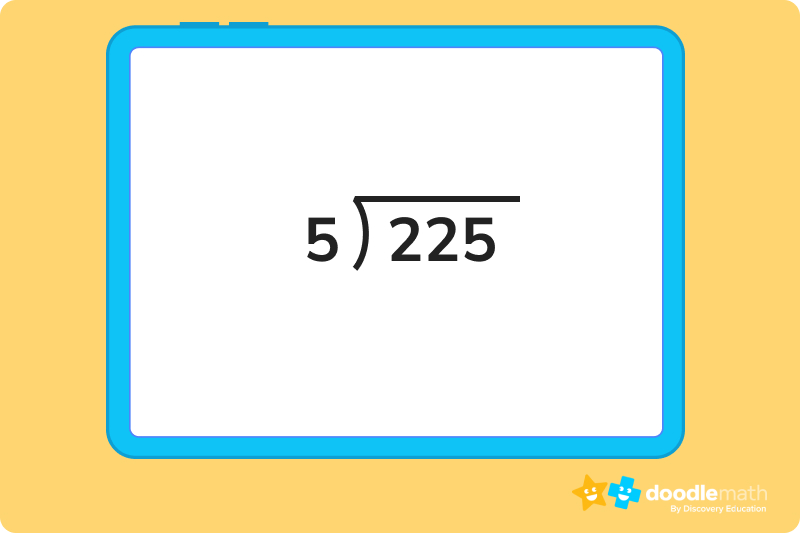### Step 2:Now, let’s take a look at the first number from the left in our divisor. Since 2 is less than 5, the dividend, we know we can’t do any division here. So, we write a 0 as our first number in the quotient and multiply it by our 5, giving us a product of 0.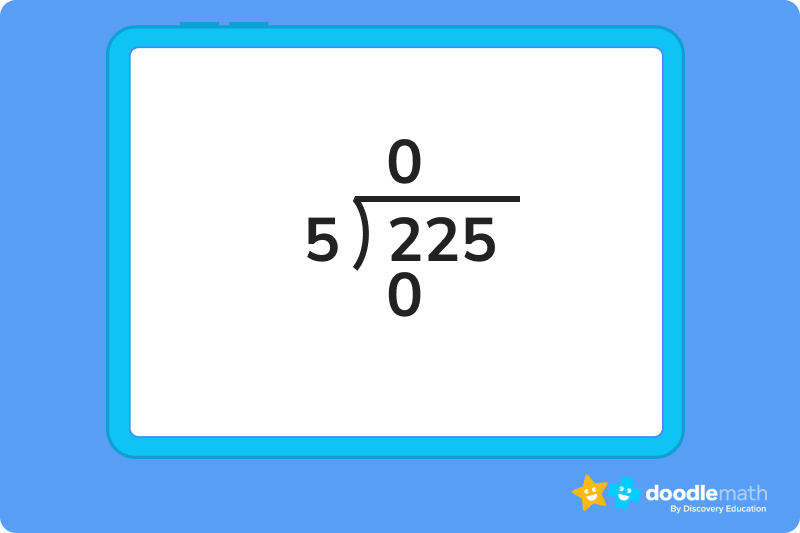### Step 3:We then need to subtract this 0 from the first digit of the dividend, 2. Now we can drag the 2 in the tens place down and place it next to the difference we calculated in step 2. This gives us a value of 22.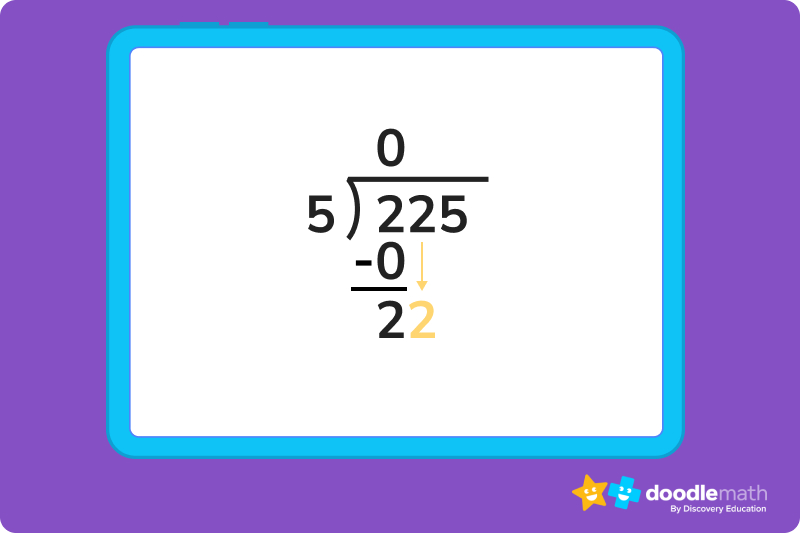### Step 4:22 isn’t exactly divisible by 5, but we know 5 x 4 = 20, or that 5 fits into 20 four times. That means we can write a four in the quotient to the right of our 0. We then write that 20 below our 22, and subtract.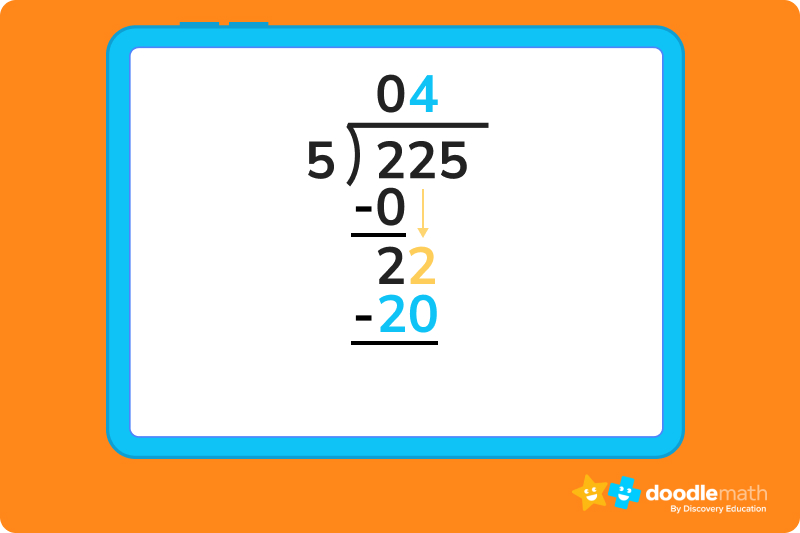### Step 5:Our subtraction from step 4 gives us a value of 2. Now we drag the 5 in the ones place of 225 all the way down next to that 2, giving us a value of 25.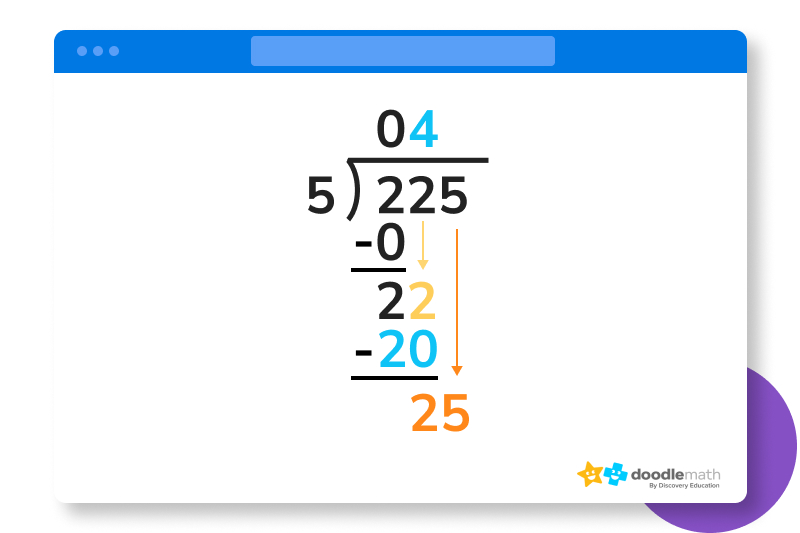### Step 6:Now, we can divide that final 25 by our original dividend of five. 25 divided by 5 equals 5, which we write in the final place of our quotient.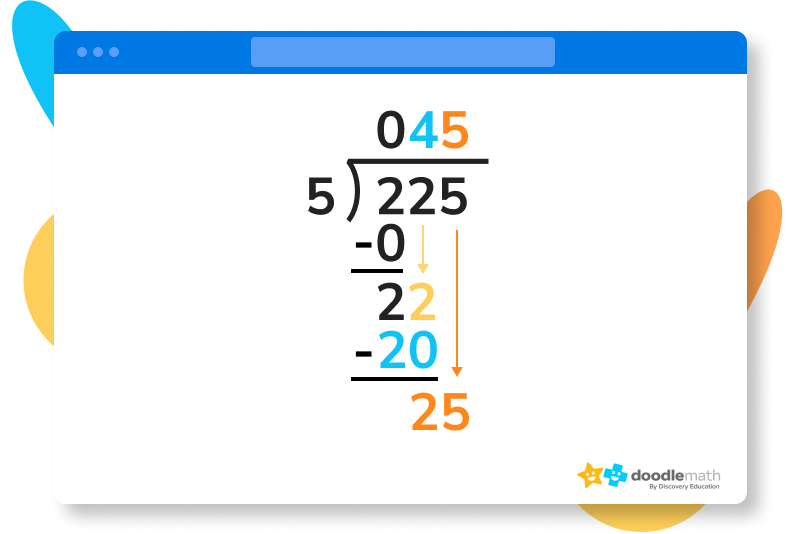## Let’s practice together!#### Solve 135 ÷ 6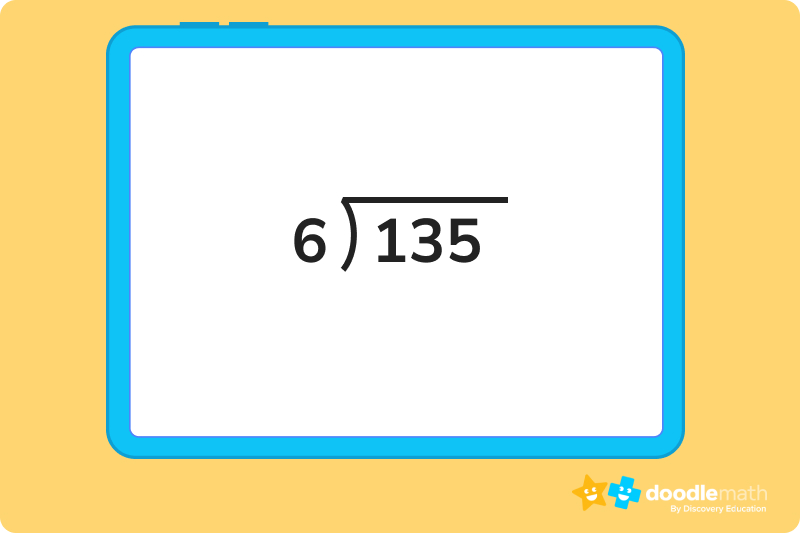1. Since we know 1 is less than 6, we can write a 0 as the first number in our quotient, and drag our 1 down.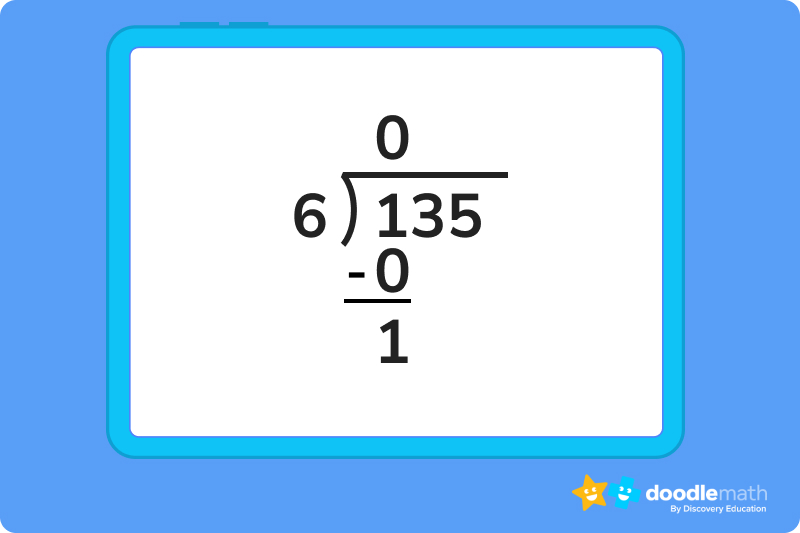2. We then drag the 3 down next to our 1, giving us a value of 13. Since 6 fits into 13 twice, 6 x 2 = 12, we can write that 2 next to our 0 in the quotient.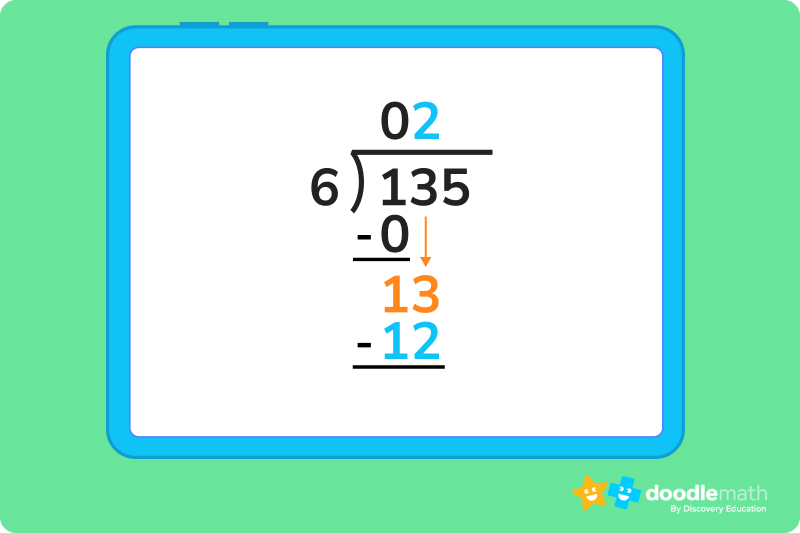3. We then subtract 12 from 13, giving us a 1.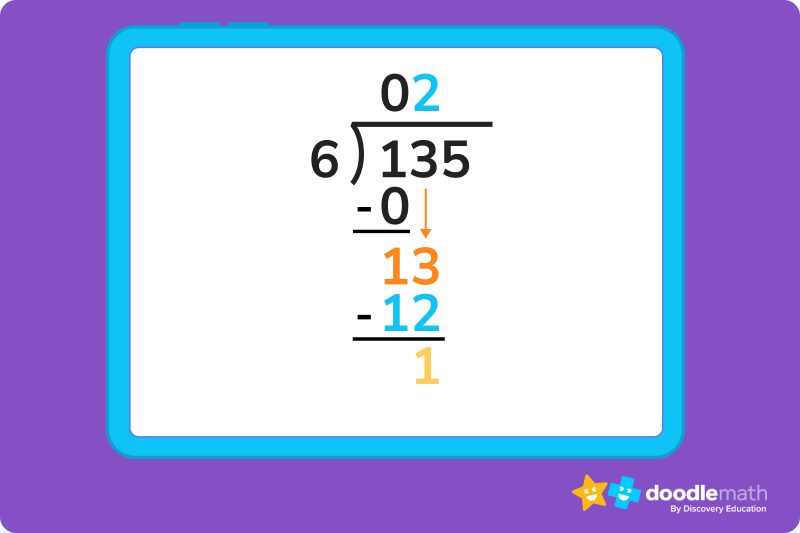4. Now we can drag our 5 down and place it next to that one, giving us 15. Six fits into 15 twice, meaning we can write another two in our quotient.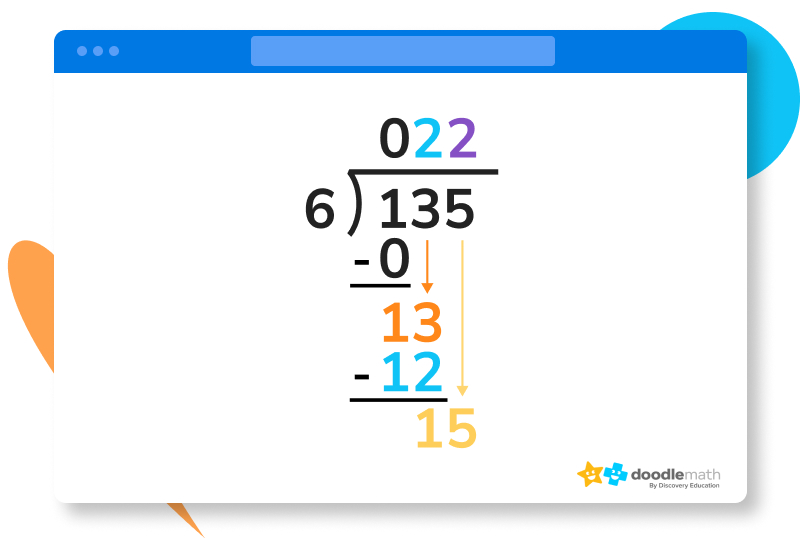5. However, 15 – 12 = 3, and there are no more numbers to drag down! Here is where we use the term REMAINDER. 3 is the remainder here, since there is no more possible division to perform. Our final answer is 22 with a remainder of 3.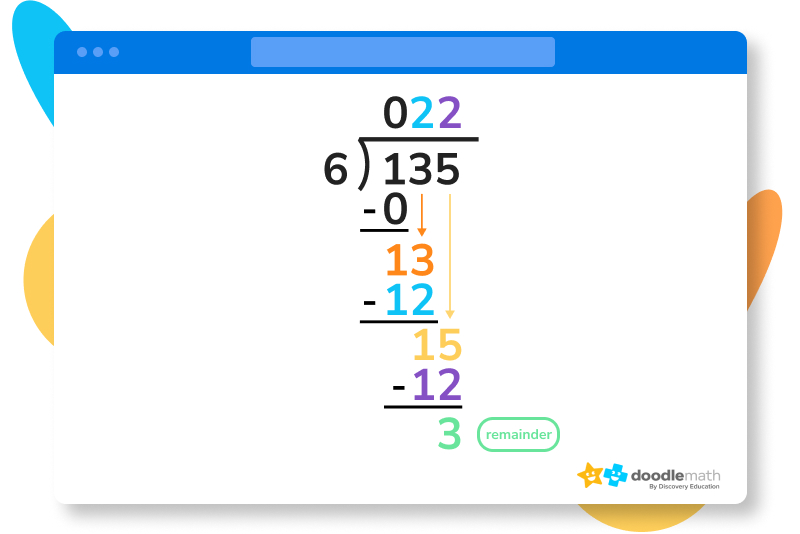#### Solve 339 ÷ 3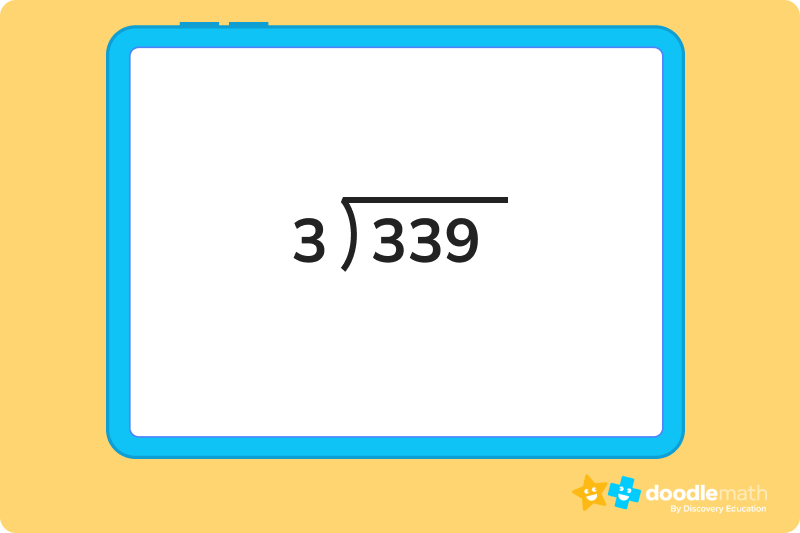1. The 3 in the hundreds place of the dividend is divisible by 3, giving us a value of 1 to write first in the quotient.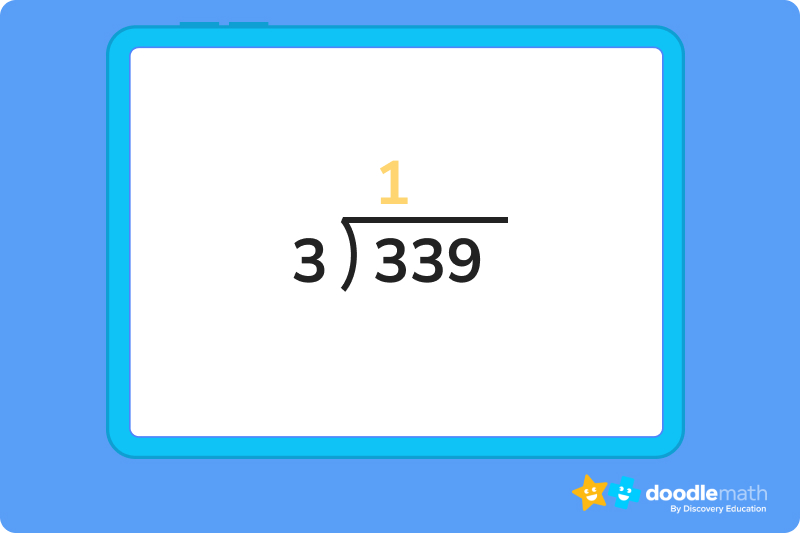2. Now, we can write a 3 underneath the 3 in the hundreds place, and subtract. This should give us a value of 0.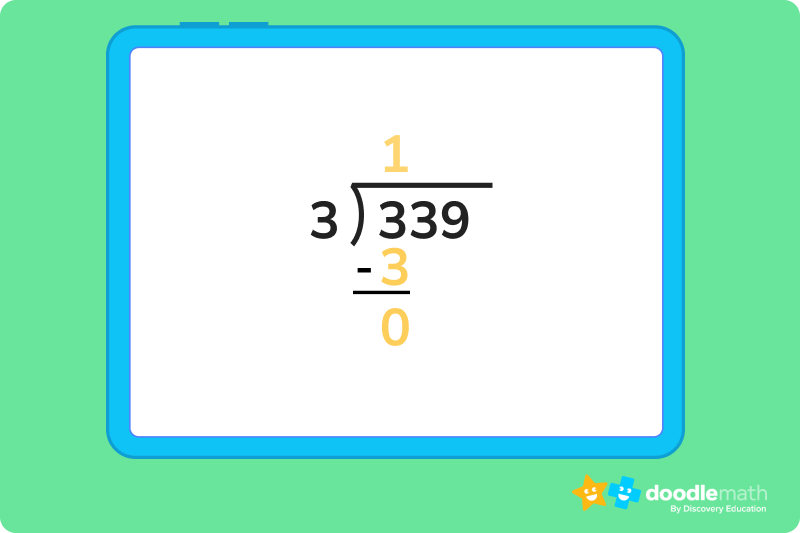3. We can then drag the three in the tens place down next to our 0.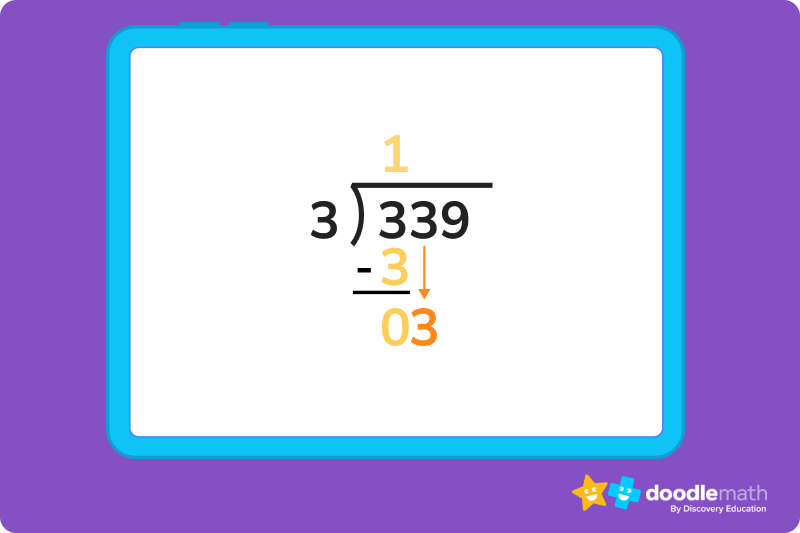4. Let’s repeat the same process in step 1, since 3 is still divisible by 3. This will give us another 1 in the quotient, and a subtraction equation of 3 – 3 that gives another 0.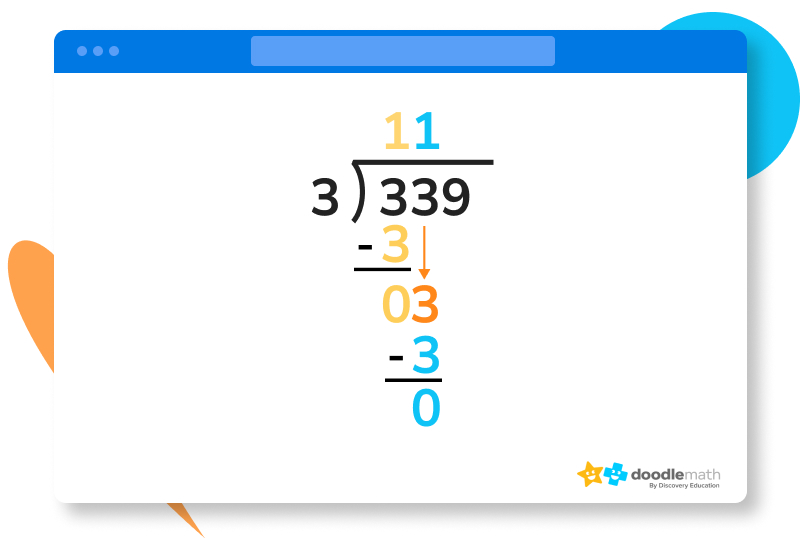5. Now, let’s drag the 9 down next to our new 0. 9 is also divisible by 3. 93 = 3, meaning we can write a 3 as our last digit in our quotient. 3-3=0, meaning we’ve finished our long division. We should have a value of 113 in our quotient.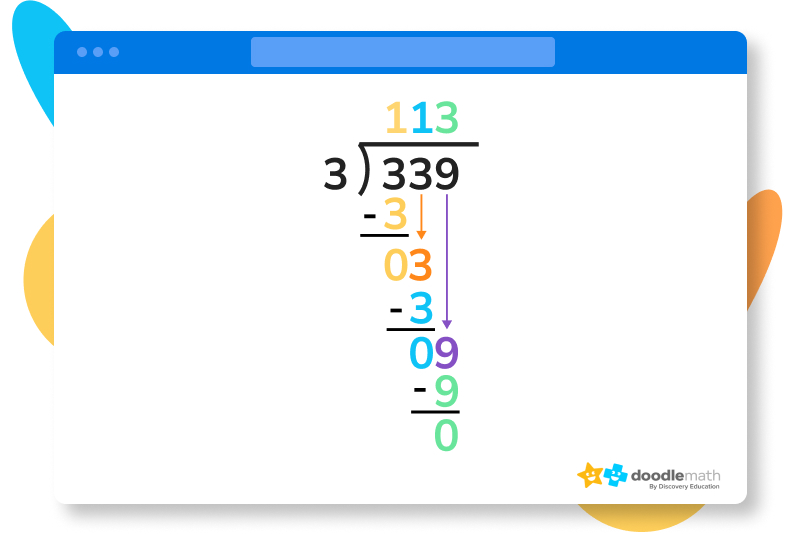Ready to give it a go?

Now it’s your turn to put your long division skills to the test. Use your knowledge of long division to solve the following problems. Feel free to consult the guide if you get stuck.

## Practice problems

The answer is 71 with a remainder of 6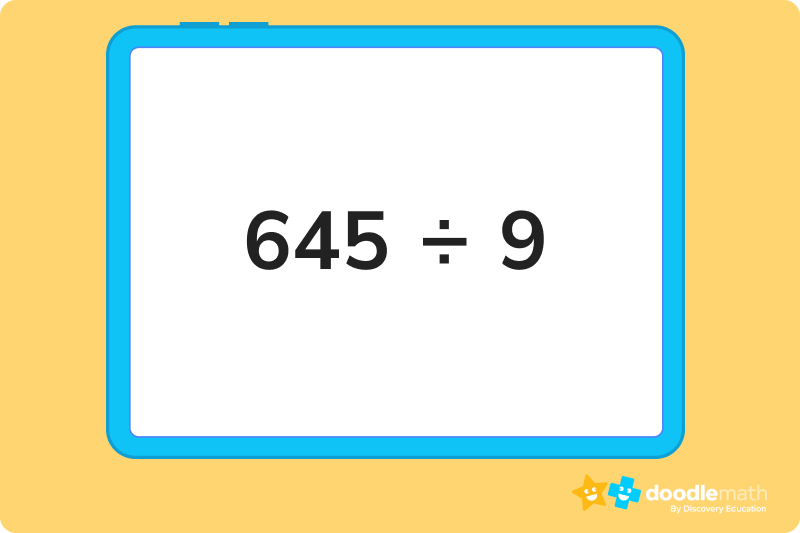The answer is 52 with a remainder of 6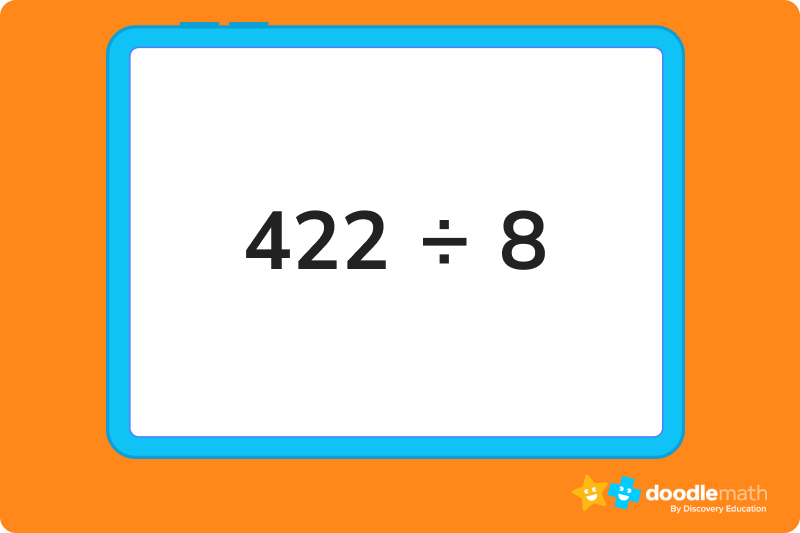The answer is 54 with a remainder of 2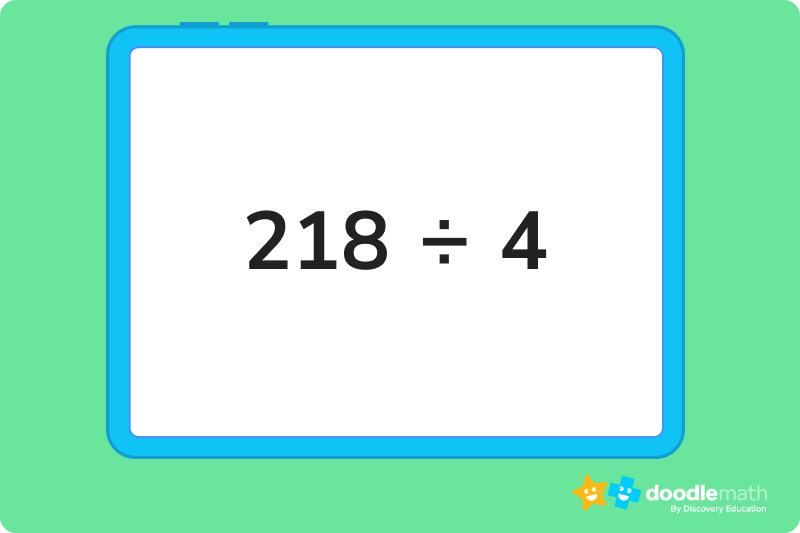## Parent Guide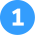The answer is 71 with a remainder of 6

How did we get here?

1. 6 is smaller than 9, meaning we write a 0 in the quotient, and subtract that 0 from our 6.
2. 6 – 0 = 6, and we can drag our next digit, 4, down to create a value of 64.
3. 64 isn’t divisible by 9, but 9 x 7 = 63. That means we can fit seven values of 9 into 64, so we should write a 7 next to our 0 in the quotient.
4. 64 – 63 = 1, and we can drag our final digit 5 down next to this 1 to give us 15.
5. 9 fits into 15 once, so we write a 1 in the quotient and subtract 9 from 15, giving us 6.
6. Since 6 is less than 9, we know we can stop our division, giving us a quotient of 71 with a remainder of 6.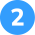The answer is 52 with a remainder of 6

How did we get here?

1. 4 is less than 8, so we need to write a 0 in our quotient and subtract this 0 from our 4. 4 – 0 will give us 4.
2. Now we can drag our 2 down next to this 4, giving us a value of 42. 8 fits into 42 only 5 times (8 x 5 = 40), so we should write a 5 in our quotient.
3. We then subtract 40 from 42, giving us 2. Now we drag the 2 in the ones place of our dividend down, giving us 22.
4. 8 fits into 22 twice, meaning we write a two in the quotient and subtract 16 from 22.
5. 22 – 16 = 6, so we are left with a quotient of 52 and a remainder of 6.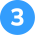The answer is 54 with a remainder of 2

How did we get here?

1. 2 is smaller than 4, so we write a 0 in the quotient. We then multiply 2 by 0, and subtract that value from the 2 in the dividend.
2. We can then drag the 1 in the tens place down next to this 2 to give us 21.
3. 4 fits into 21 five times (4 x 5 = 20), so we write a 5 in the quotient and subtract 20 from 21.
4. This gives us a value of one, and then drag our final value of 8 down to give us 18. 4 fits into 18 four times, so we write a 4 in the quotient.
5. 18 – 16 = 2, so we are left with a quotient of 54 with a remainder of 2.

## FAQs about math strategies for kidsWe understand that diving into new information can sometimes be overwhelming, and questions often arise. That’s why we’ve meticulously crafted these FAQs, based on real questions from students and parents. We’ve got you covered!

Just remember to divide, multiply, subtract, bring that next number down, and keep repeating that process. Long division is all about repeating the same steps over and over again until you can’t do any more division.

The hardest part is remembering to drag that next digit down so you can repeat the process. But if you can master those basic steps, you’ll begin to build some muscle memory that makes the process much easier.

For these problems, we check the first two digits of the dividend and see if they are divisible by our divisor. If the first two digits of the dividend are less than the divisor, we’ll need to consider the first three digits of the dividend instead. Now we can follow the same steps. Figure out how many times we can multiply our divisor into our dividend, and subtract that value.

Long division is often more accurate than shorthand division, because the steps are written out plainly in front of us. This allows us to track our division through a step-by-step process, and continue dividing until we discover an exact remainder. Think of long division as the most convenient and accurate way of dividing larger numbers. As you continue to learn mathematics, it’ll become an essential skill for solving more complicated problems.

This process might seem a bit more complicated, but it relies on the same rules and procedures we’ve been using this whole time. Since we know that the quotient, remainder and divisor are all related to the dividend in some way, there is a mathematical procedure we can use to calculate each. To find the dividend, simply multiply the quotient by the divisor, and add that product to your remainder.## Related Posts

Title 1

Title 2

Title 3

Lesson credits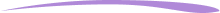Taylor Hartley

Taylor Hartley is an author and an English teacher based in Charlotte, North Carolina. When she's not writing, you can find her on the rowing machine or lost in a good novel.

Jill Padfield has 7 years of experience teaching high school mathematics, ranging from Alegra 1 to AP Calculas. She is currently working as a Business Analyst, working to improve services for Veterans while earning a masters degree in business administration.

Taylor Hartley

Taylor Hartley is an author and an English teacher based in Charlotte, North Carolina. When she's not writing, you can find her on the rowing machine or lost in a good novel.

Jill Padfield has 7 years of experience teaching high school mathematics, ranging from Alegra 1 to AP Calculas. She is currently working as a Business Analyst, working to improve services for Veterans while earning a masters degree in business administration.# Are you a parent, teacher or student?

Are you a parent or teacher?

## Hi there!

Book a chat with our team